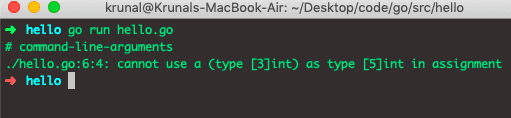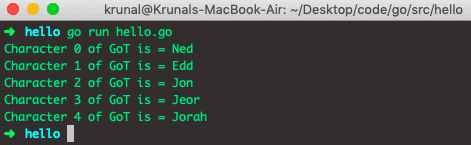# Golang Array: The Complete Guide

Golang programming language provides an array that is a fixed-size collection of elements of the same type.

## Golang Array

A Golang array is a built-in data structure that can store the fixed-size sequential collection of items of the same type. The elements of an array are stored sequentially and can be accessed using their index. If we want

The array is used to store data collection, but it is often more useful to think of the array as the collection of variables of the same type.

Instead of declaring individual variables, such as no1, no2, …, and no99, you declare one array variable, such as numbers, and use no, no, and …, no to represent individual variables.

## How to declare an array in Golang

To declare an array in Golang, specify the type of elements and the number of items required by an array. An array belongs to type n[T]. The n denotes the number of elements in an array, and T represents the type of each element. Thus, the number of items n is also a part of the kind.

There are different ways to declare arrays. Let’s look at them one by one.

`var a[n]T`

Here is how you can declare the array of 5 integers.

`var aint`

See the following code example.

```// hello.go

package main

import (
"fmt"
)

func main() {
var a int //int array with length 5
fmt.Println(a)
}
```

See the below output.The var an int declares an integer array of length 5. All elements in the array are automatically assigned a zero value of the array type.

In this case, variable a is the integer array, and hence all items of a are assigned to 0, the zero value of the integer.

An array index starts from 0 and ends with length – 1. Let’s assign some values to the above array.

```// hello.go

package main

import "fmt"

func main() {
var a int
a = 18
a = 19
a = 21
a = 29
a = 46
fmt.Println(a)
}
```

## Shorthand Declaration of Array in Golang

Let’s create the same array using the shorthand declaration. See the below code.

```// hello.go

package main

import "fmt"

func main() {
a := int{18, 19, 21, 29, 46} // short hand declaration to create array
fmt.Println(a)
}
```

It will give us the same output as the above program.It is not necessary that all items in the array have to be assigned a value during the shorthand declaration. See the below scenario.

```// hello.go

package main

import "fmt"

func main() {
a := int{18, 19, 21} // short hand declaration to create array
fmt.Println(a)
}
```

In the above code, we have defined array 5 but declared only three items.

In this case, the remaining items have 0 values by default. For example, see the output of the above code.## Array’s length is part of its type.

The size of an array is a part of the type. Hence int and int are distinct types. Because of this, arrays cannot be resized.

Don’t worry about the restriction because slices exist to overcome this disadvantage. However, that means that you cannot resize the array because it would mean changing its type, and you are not allowed to change the type of a variable in Golang.

See the below code to understand better.

```package main

// hello.go

func main() {
a := int{5, 78, 8}
var b int
b = a
}
```

If you are using Visual Studio Code and have installed all the Go extensions, you might get an error inside the VSCode editor. Now, if you run the above code, then it will throw an error.So, in the above code, variables a and b have different array sizes.

That is why we can not assign each other due to the array length difference, which is strictly prohibited in Golang.

## Arrays in Golang are value types.

Arrays in Golang are value types, unlike other languages like C, C++, Python, and Java, where arrays are reference types.

That means that when you assign the array to the new variable or pass the array to the function, the entire array is copied.

So if you want to make any changes to the copied array, the original array won’t be affected and will remain unchanged. See the below code for understanding.

```// hello.go

package main

import "fmt"

func main() {
arrA := string{"Ned", "Edd", "Jon", "Jeor", "Jorah"}
arrB := arrA

arrB = "Gendry"

fmt.Println("array A = ", arrA)
fmt.Println("array B = ", arrB)
}
```

See the output.In the above code, we have copied the content of arrA to arrB, then we have modified the arrB, but still, arrA remains the same, and it does not change.

## How to find array length in Golang

To get a length of an array in Golang, use the len() function. The len() is a built-in Golang function that accepts an array as a parameter and returns the array’s length.

```// hello.go

package main

import "fmt"

func main() {
data := [...]string{"Ned", "Edd", "Jon", "Jeor", "Jorah"}
fmt.Println("length of data is", len(data))
}
```

See the below output.## How to iterate an array in Golang

To iterate an array in Golang, use the for loop.

```// hello.go

package main

import "fmt"

func main() {
data := [...]string{"Ned", "Edd", "Jon", "Jeor", "Jorah"}
for i := 0; i < len(data); i++ { //looping from 0 to the length of the array
fmt.Printf("%d th element of data is %s\n", i, data[i])
}
}
```

See the below output.## Iterating over an array using a range operator

Golang provides a more robust form of for loop using the range operator.

Here’s how you can use the range operator with for loop to iterate over the array.

```// hello.go

package main

import "fmt"

func main() {
data := [...]string{"Ned", "Edd", "Jon", "Jeor", "Jorah"}
for index, value := range data {
fmt.Printf("Character %d of GoT is = %s\n", index, value)
}
}```

See the below output.## Multidimensional arrays in Golang

Till now, we have dealt with only a one-dimensional array. However, you can also create multi-dimensional arrays in Golang.

The following example demonstrates how to create multidimensional arrays.

```// hello.go

package main

import "fmt"

func main() {
multiA := int{
{19, 21},
{29, 46},
}
fmt.Println(multiA)
}
```

See the below code.That’s it for arrays. An array’s disadvantage is that it comes with the restriction that the array’s length is fixed in Go. Thus, it is impossible to increase the length of the array. This is how the Golang slices come into the picture.

In the Go language, slices are more common practices in programming than conventional arrays.

That’s it for this tutorial.

## Recommended Posts

Golang slice

Golang struct

Golang Function

Golang Slice Append

Categories Go

This site uses Akismet to reduce spam. Learn how your comment data is processed.